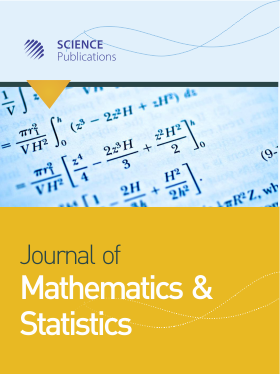Frequency: Continuous
ISSN: 1549-3644 (Print)
ISSN: 1558-6359 (Online)
Research Article Open Access

# Bayesian Analysis of Longitudinal Ordinal Data Using Non-Identifiable Multivariate Probit Models

Xiao Zhang1
• 1 Department of Mathematics, Michigan Technological University, United States

## Abstract

Multivariate probit models have been explored foranalyzing longitudinal ordinal data. However, the inherent identification issuein multivariate probit models requires the covariance matrix of the underlyinglatent multivariate normal variables to be a correlation matrix and thushinders the development of efficient Bayesian sampling methods. It is knownthat non-identifiable models may produce Markov Chain Monte Carlo (MCMC)samplers with better convergence and mixing than identifiable models.Therefore, we were motivated to construct a non-identifiable multivariateprobit model and to develop efficient MCMC sampling algorithms. In comparisonwith the MCMC sampling algorithm based on the identifiable multivariate probitmodel, which requires a Metropolis-Hastings (MH) algorithm for sampling acorrelation matrix, our proposed MCMC sampling algorithms based on thenon-identifiable model circumvent an MH algorithm by a Gibbs sampler forsampling a covariance matrix and thus accelerate the MCMC convergence. Weillustrate our proposed methods using simulation studies and two real dataapplications. Both the simulation studies andthe real data applications show that constructingnonidentifiable models may improve the convergence of the MCMC algorithmscompared with the identifiable models. The marginalization of the redundantparameters in the non-identifiable models should be considered in developingefficient MCMC sampling algorithms. This investigation shows that constructionof non-identifiable models is valuable in developing MCMC sampling methods and illustratesadvantages and disadvantages of construction of non-identifiable models toimprove the convergence of the MCMC sampling components.

Journal of Mathematics and Statistics
Volume 18 No. 1, 2022, 163-175

DOI:

Submitted On: 27 April 2022 Published On: 14 November 2022

How to Cite: Zhang, X. (2022). Bayesian Analysis of Longitudinal Ordinal Data Using Non-Identifiable Multivariate Probit Models. Journal of Mathematics and Statistics, 18(1), 163-175. https://doi.org/10.3844/jmssp.2022.163.175

• 1,243 Views